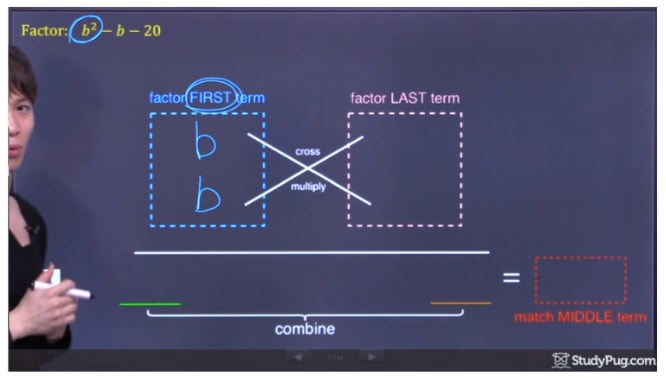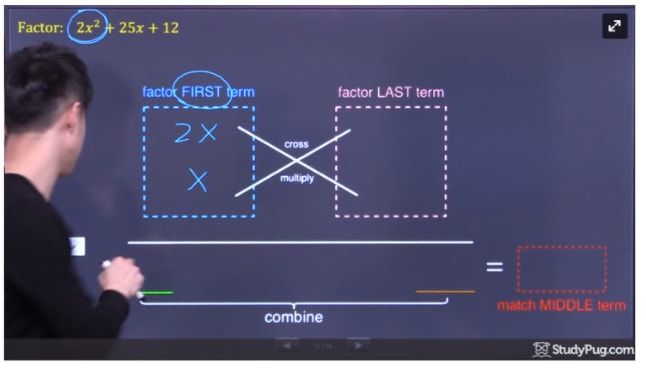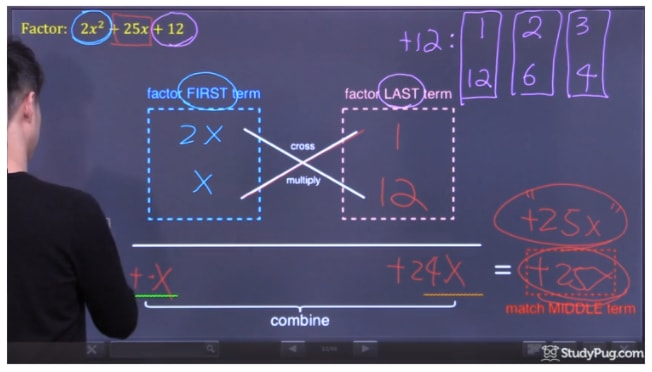# Factoring trinomials

0/1
##### Introduction
###### Lessons
1. Use "cross-multiply, then check" method to factor a trinomial
0/23
##### Examples
###### Lessons
1. Factor:
1. ${b^2} - b - 20$
2. ${x^2} - 10x + 16$
3. $2{x^3} - 14{x^2} + 24x$
4. $14 + 5y - {y^2}$
2. Factor:
1. $2{x^2} + 25x + 12$
2. $5{x^2} + 8x + 3$
3. $8{x^2} + 10x - 3$
4. $6{m^2} - 13m - 8$
5. $18{x^2} - 9x + 1$
6. $63 + 20z - 3{z^2}$
7. $8{x^2} + 8x - 6$
3. Factor:
1. $8{x^2} + xy - 9{y^2}$
2. $6{x^2} + 17xy - 3{y^2}$
3. $\frac{1}{4}{x^2} - 4xy + 16{y^2}$
4. Factor:
1. $8{x^2}{y^2} - xy - 9$
2. $25{\sin ^2}x - 35\sin x + 12$
3. $12{\cos ^2}x - 20\cos x + 3$
4. $6{\left( {x + 5} \right)^2} + 17\left( {x + 5} \right) - 3$
5. $15{\left( {x - 3} \right)^2} - 11\left( {x - 3} \right) - 14$
5. Factor:
1. ${x^6} - 17{x^3} + 30$
2. ${a^4} - 2{a^2} - 63$
3. $15{x^4} - 16{x^2} - 15$
4. $8{x^4} - 14{x^2} + 3$
###### Free to Join!
StudyPug is a learning help platform covering math and science from grade 4 all the way to second year university. Our video tutorials, unlimited practice problems, and step-by-step explanations provide you or your child with all the help you need to master concepts. On top of that, it's fun - with achievements, customizable avatars, and awards to keep you motivated.
• #### Easily See Your ProgressWe track the progress you've made on a topic so you know what you've done. From the course view you can easily see what topics have what and the progress you've made on them. Fill the rings to completely master that section or mouse over the icon to see more details.
• #### Make Use of Our Learning Aids###### Practice Accuracy

See how well your practice sessions are going over time.

Stay on track with our daily recommendations.

• #### Earn Achievements as You LearnMake the most of your time as you use StudyPug to help you achieve your goals. Earn fun little badges the more you watch, practice, and use our service.
• #### Create and Customize Your AvatarPlay with our fun little avatar builder to create and customize your own avatar on StudyPug. Choose your face, eye colour, hair colour and style, and background. Unlock more options the more you use StudyPug.

## How to factor trinomials

Trinomials are algebraic expressions that has three terms in it. Quadratic trinomials are in the form of a${x^2}$ + bx + c, and the a, b, and c all stands for a number.

In order to factor trinomials, you'll have to work to find two numbers that will multiply to equal the "c" from the quadratic form above, and also add up to equal "b". These are the steps for the easier questions where the first "a" is equalled to 1. For harder problems, "a" will be a number that is not one. You'll have to first multiply "a" and "c", and then find factors of the product "a*c" that also add up to "b".

We'll explore this with example questions to demonstrate how to factor trinomials.

Question 1

Factor: ${b^2} - b - 20$

Solution:

In this example, we are going to use the "cross-multiply, then check" method to factor the trinomial. This is one of the ways to factor trinomials.

Factor the first term ${b^2}$, put the results (b and b)  in the first box.Factor the term b^2 and put them into the first box

Factor the last term -20. There are a few combinations (1x20, 2x10, 4x5) that can give us 20, so which one is it? We'll cross-multiply those combinations to the factors of the first term. See which combination will produce an answer that matches the middle term (in this question, the middle term is –b).

Out of those combinations, 4x-5 (which equals -1) is able to produce the matching middle term -b.

After all the steps, we successfully factored the trinomial  ${b^2} - b - 20$ into $\left( {b + 4} \right) \bullet \left( {b - 5} \right)$

Question 2:

Factor: $2{x^2} + 25x + 12$

Solution:

The "cross-multiply, then check" method can also be used on harder trinomials in which the leading coefficient is not 1. In this question, the leading coefficient is 2 (from the leading term $2{x^2}$).

Now, we factor the first term $2{x^2}$. The result is 2x and x. we put them in the first boxFactor the first term 2x^2 and put into the first box

In order to factor the last term +12, there are a few combinations (1x12, 2x6, 3x4). We'll once again cross-multiply those combinations to the factors of the first term. See which combination will produce a matching middle term (in this question, the middle term is +25x)

Out of those combinations, 1x12 is able to produce the matching middle term +25x. That is because (2x x 12) + (x x 1) = 24x + x = 25xFind out that 12 and 1 match the middle term of 25x

After all the steps, we successfully factored the trinomial  $2{x^2} + 25x + 12$ into $\left( {2x + 1} \right) \bullet \left( {x + 12} \right)$